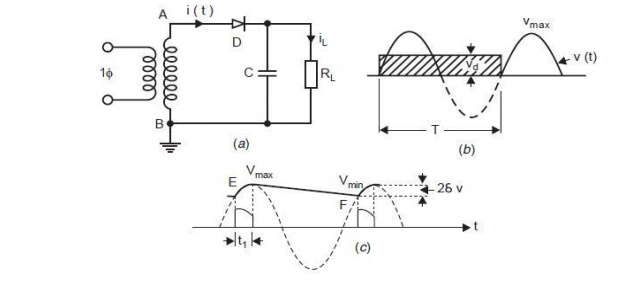Home | | High Voltage Engineering | Half Wave Rectifier Circuit - Generation of High Voltages and Currents

# Half Wave Rectifier Circuit - Generation of High Voltages and CurrentsThe simplest circuit for generation of high direct voltage is the half wave rectifier shown in Fig. 3.1 Here RL is the load resistance and C the capacitance to smoothen the dace. output voltage.

Half Wave Rectifier Circuit

The simplest circuit for generation of high direct voltage is the half wave rectifier shown in Fig. 3.1 Here RL is the load resistance and C the capacitance to smoothen the dace. output voltage. If the capacitor is not connected, pulsating dace. voltage is obtained at the output terminals where as with the capacitance C, the pulsation at the output terminal are reduced. Assuming the ideal transformer and small internal resistance of the diode during conduction the capacitor C is charged to the maximum voltage Vmax during conduction of the diode D. Assuming that there is no load connected, the dace. Voltage across capacitance remains constant at Vmax whereas the supply voltage oscillates between ± Vmax and during negative half cycle the potential of point A becomes – Vmax and hence the diode must be rated for 2Vmax. This would also be the case if the transformer is grounded at A instead of B as shown in Fig. 3.1 (a). Such a circuit is known as voltage doublers due to Villard for which the output voltage would be taken across D. This dace. voltage, however, oscillates between zero and 2Vmax and is needed for the Cascade circuit.Figure: 3.1 (a) Single Phase rectifier (b) Output voltage without C (c) Output voltage with Cuff the circuit is loaded, the output voltage does not remain constant at Vmax. After point E (Fig.3.1 (c)), the supply voltage becomes less than the capacitor voltage, diode stops conducting.

The capacitor cannot discharge back into the a.c. system because of one way action of the diode. Instead, the current now flows out of C to furnish the current ill through the load. While giving up this energy, the capacitor voltage also decreases at a rate depending on the time constant CR of the circuit and it reaches the point F corresponding to V min. Beyond F, the supply voltage is greater than the capacitor voltage and hence the diode D starts conducting charging the capacitor C again to Vmax and also during this period it supplies current to the load also. This second pulse of imp(ic + il) is of shorter duration than the initial charging pulse as it serve mainly to restore into C the energy that C meanwhile had supplied to load. Thus, while each pulse of diode current lasts much less than a half cycle, the load receives current more continuously from C. Assuming the charge supplied by the transformer to the load during the conduction period t, which is very small to be negligible, the charge supplied by the transformer to the capacitor during conduction equals the charge supplied by the capacitor to the load. Note that ic>> iL. During one period T = 1/f of the a.c voltage, a charge Q is transferred to the load RL and is given aswhere I is the mean value of the dace output iL(t) and VRL(t) the dace. voltage which includes a ripple as shown in Fig. 2.1 (c). This charge is supplied by the capacitor over the period T when the voltage changes from Vmax to Vmin over approximately period T neglecting the conduction period of the diode. Suppose at any time the voltage of the capacitor is V and it decreases by an amount of dV over the time dt then charge delivered by the capacitor during this time isTherefore, if voltage changes from Vmax to Vmin, the charge delivered by the capacitorOr the magnitude of charge delivered by the capacitorThe above equation shows that the ripple in a rectifier output depends upon the load current and the circuit parameter like f and C. The product fC is, therefore, an important design factor for the rectifiers. The higher the frequency of supply and larger the value of filtering capacitor the smaller will be the ripple in the dace. output. The single phase half-wave rectifier circuits have the following disadvantages:

·        The size of the circuits is very large if high and pure dace. output voltages are desired.

·        The h.t. transformer may get saturated if the amplitude of direct current is comparable with the nominal alternating current of the transformer.

It is to be noted that all the circuits considered here are able to supply relatively low currents and therefore are not suitable for high current applications such as HVDC transmission. When high dace. voltages are to be generated, voltage doubler or cascaded voltage multiplier circuits are used. One of the most popular doubler circuit due to Greinacher is shown in Fig. 3.2. Suppose B is more positive with respect to A and the diode D1 conducts thus charging the capacitor C1 to Vmax with polarity as shown in Fig. 3.2. During the next half cycle terminal A of the capacitor C1 rises to Vmax and hence terminal M attains a potential of 2 Vmax. Thus, the capacitor C2 is charged to 2 Vmax through D2. Normally the voltage across the load will be less than 2 Vmax depending upon the time constant of the circuit C2RL.

Study Material, Lecturing Notes, Assignment, Reference, Wiki description explanation, brief detail

Related Topics